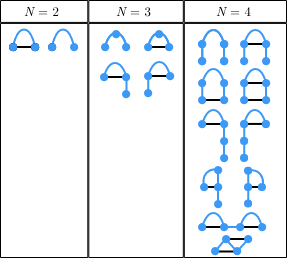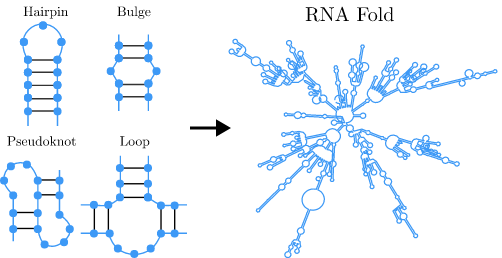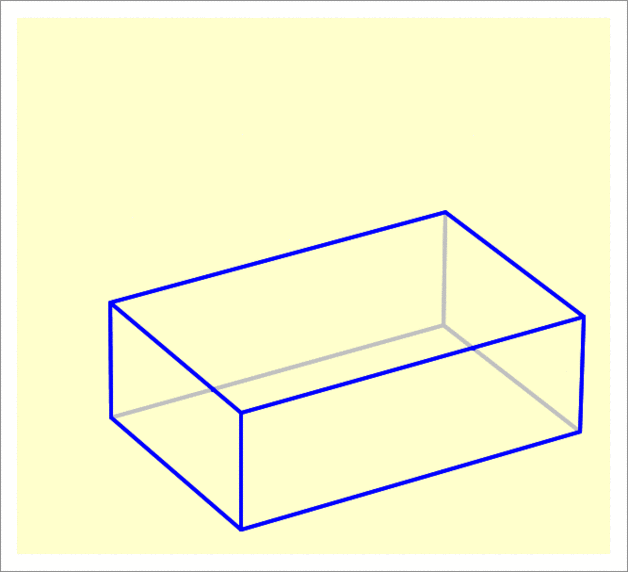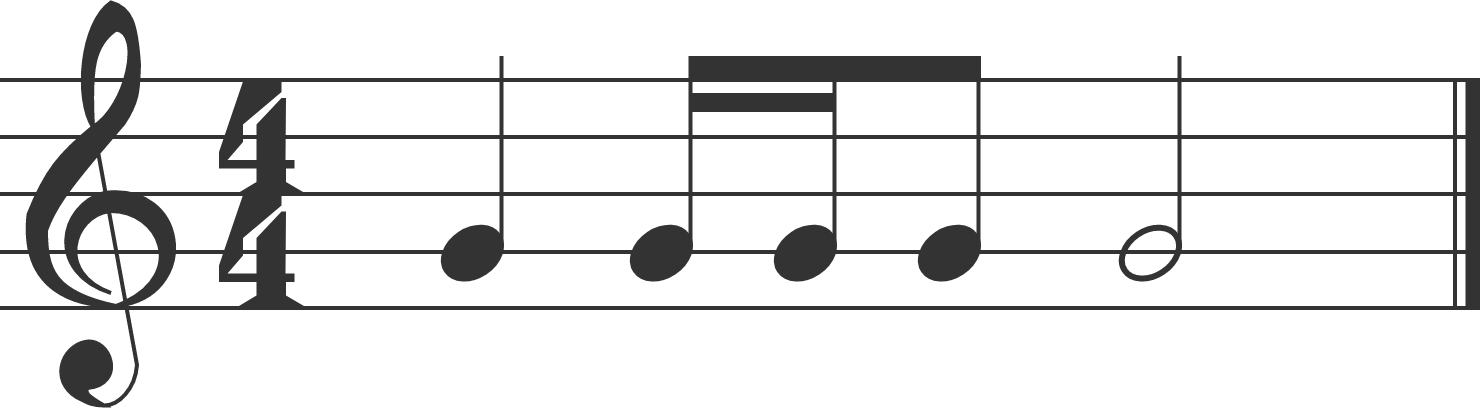# Problems of the Week

Contribute a problemJane, Kate, and Lily are perfect logicians and always tell the truth.

They are each wearing a hat marked with a distinct positive integer. They know that one of the hats has a single-digit integer, $N,$ written on it, and that the other two hats each have a divisor of $N$ written on them. Each person can only see the two numbers on the others’ hats.

• Jane: "I don't know my number."
• Kate: "I don't know my number."
• Lily: "But I know my number!"
• Kate: "Now I know my number, too!"
• Jane: "I still don't know my number."

What is the sum of the numbers written on Kate and Lily’s hats?RNA molecules are long chains of different repeating units called bases, which can either fold and pair with any other base on the same molecule, or remain unpaired. For short RNA chains, the number of possible arrangements can be quickly enumerated by inspection. Which arrangement the RNA tends to fold into depends on its sequence of bases: given a sequence, every arrangement can be "scored", as some pairs are more likely to form stable bonds than others.

For biological RNAs, the typical length is greater than 100 bases. As with shorter lengths, the arrangement that the RNA folds into depends on its sequence, but the number of possible arrangements to consider is outrageously large, including many common structural motifs shown below.

For an RNA molecule consisting of $N=100$ bases, there are $n$ different arrangements which it can choose from.

How many digits are in $n$ expressed in base $10?$You need to be connected to run code

A cuboid has base dimensions $30 \times 20$ and a height of $10$ and is lying flat on the $xy$-plane.

It undergoes two rotations—the first is $30^{\circ}$ about the edge measuring $20$, and the second is also $30^{\circ}$ about the edge measuring $30$. Both rotations are such that the center of the cuboid is raised upward.

Find the $z$-coordinate (that is, the altitude) of the highest point of the cuboid after applying the two rotations (to 2 decimal places).$f(x)=\overbrace{\sin\sin ... \sin}^{70\text{ sines}} (x).$

Calculate the seventh derivative of $f$ evaluated at $x=0.$

Note: Using computer software to answer this question is an even deadlier sin than nesting 70 sines inside each other, so don't do it.

In the common time meter, one bar of music consists of note values equivalent to $4$ quarter notes. Using the note value system, $1$ whole note is equivalent to $2$ half notes, $1$ half note is equivalent to $2$ quarter notes, $1$ quarter note is equivalent to $2$ eighth notes, and $1$ eighth note is equivalent to $2$ sixteenth notes. (For our British friends, a whole note is a semibreve, a half note is a minim, a quarter note is a crotchet, an eighth note is a quaver, and a sixteenth note is a semiquaver.)

An example of a rhythm in one bar of common time meter with $1$ quarter note, $2$ sixteenth notes, $1$ eighth note, and $1$ half note is shown below.Using only whole notes, half notes, quarter notes, eighth notes, and sixteenth notes (with no rests or dotted modifiers), how many unique rhythms can be made in one bar of common time meter?

×